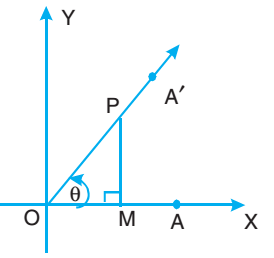# Trigonometric Identities

When equation involving a variable is true for all values of the variable, it is called an identity.

Let XOX′ and YOY′ be the rectangular axes. Let A be any point on OX. Let the ray OA start rotating in the plane in an anti-clockwise direction about the point O till it reaches the final position OA′ after some interval of time. Let ∠A′OA = θ. Take any point P on the ray OA′. Draw PM ⊥ OX.In right angled ΔPMO,

$$\sin \theta = \frac{PM}{OP}$$

$$\cos θ = \frac{OM}{OP}$$

$$\sin^2 \theta + \cos^2 \theta = \left( \frac{PM}{OP} \right)^2 + \left( \frac{OM}{OP} \right) ^2$$

$$= \frac{PM^2 + OM^2}{OP^2}$$

$$= \frac{OP^2}{OP^2}$$

$$= 1$$

### Identities

$$\sin^2 \theta + \cos^2 \theta = 1$$

$$\sec^2 \theta - \tan^2 \theta = 1 \text{ or } \sec^2 \theta = 1 + \tan^2 \theta$$

$$\csc^2 \theta - \cot^2 \theta = 1 \text{ or } \csc^2 \theta = 1 + \cot^2 \theta$$

### Method to Solve Questions on Trigonometric identities

Step 1: Choose L.H.S. or R.H.S., whichever looks to be easy to simplify.

Step 2: Use different identities to simplify the L.H.S. (or R.H.S.) and arrive at the result on the other hand side.

Step 3: If you don’t get the result on R.H.S. (or L.H.S.) arrive at an appropriate result and then simplify the other side to get the result already obtained.

Step 4: As both sides of the identity have been proved to be equal the identity is established.